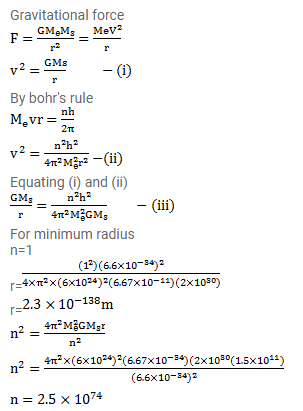# The earth resolves round the sun due to gravitational attraction.

Question:

The earth resolves round the sun due to gravitational attraction. Suppose that the sun and the earth are point particles with their existing masses and that Bohr's quantization rule for angular momentum is valid in the case of gravitation. Calculate the minimum radius the earth can have for its orbit.

What is the value of the principal quantum number $n$ for the present radius? Mass of the earth $=6.0 \times 10^{24} \mathrm{~kg}$, mass of the sun $=2.0 \times 10^{20}$, earth - sun distance $=1.5 \times 10^{11} \mathrm{~m}$.

Solution: